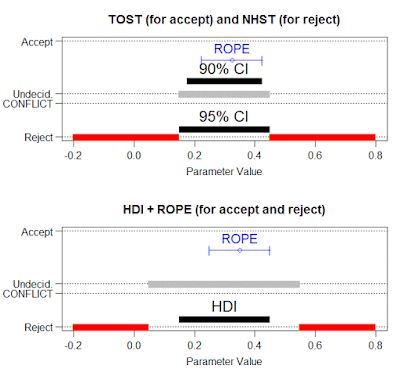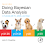## Thursday, February 16, 2017

### Equivalence testing (two one-sided test) and NHST compared with HDI and ROPE

In this blog post I show that frequentist equivalence testing (using the procedure of two one-sided tests: TOST) with null hypothesis significance testing (NHST) can produce conflicting decisions for the same parameter values, that is, TOST can accept the value while NHST rejects the same value. The Bayesian procedure using highest density interval (HDI) with region of practical equivalence (ROPE) never produces that conflict.

The Bayesian HDI+ROPE decision rule.

For a review of the HDI+ROPE decision rule, see this blog post and specifically this picture in that blog post. To summarize:
• A parameter value is rejected when its ROPE falls entirely outside the (95%) HDI. To "reject" a parameter value merely means that all the most credible parameter values are not practically equivalent to the rejected value. For a parameter value to be "rejected", it is not merely outside the HDI!
• A parameter value is accepted when its ROPE completely contains the (95%) HDI. To "accept" a parameter value merely means that all the most credible parameter values are practically equivalent to the accepted value. For a parameter value to be "accepted", it is not merely inside the HDI! In fact, parameter values can be "accepted" that are outside the HDI, because reject or accept depends on the ROPE.
The frequentist TOST procedure for equivalence testing.

In the frequentist TOST procedure, the analyst sets up a ROPE, and does a one-sided test for being below the high limit of the ROPE and another one-sided test for being above the low limit of the ROPE. If both limits are rejected, the parameter value is "accepted". The TOST is the same as checking that the 90% (not 95%) confidence interval falls inside the ROPE. The TOST procedure is used to decide on equivalence to a ROPE'd parameter value.

To reject a parameter value, the frequentist uses good ol' NHST. In other words, if the parameter value falls outside the 95% CI, it is rejected.

Examples comparing TOST+NHST with HDI+ROPE.

Examples below show the ranges of parameter values rejected, accepted, undecided, or conflicting (both rejected and accepted) by the two procedures.
• In these cases the ROPE is symmetric around its parameter value, with ROPE limits at $-0.1$ and $+0.1$ the central value. These are merely default ROPE limits we might use if the parameter value were the effect-size Cohen's $\delta$ because $0.1$ is half of a "small" effect size. In general, ROPE limits could be asymmetric, and should be chosen in the context of current theory and measurement abilities. The key point is that the ROPE is the same for TOST and for HDI procedures for all the examples.
• In all the examples, the 95% HDI and the 95% CI are arbitrarily set to be equal. In general, the 95% HDI and 95% CI will not be equal, especially when the CI is corrected for multiple tests or correctly computed for stopping rules that do not assume fixed sample size. But merely for simplicity of comparison, the 95% HDI and 95% CI are arbitrarily set equal to each other. The 90% CI is set to 0.83 times the width of the 95% CI, as it would be for a normal distribution. The exact numerical value does not matter for the qualitative results.
For each example, plots show the parameter values that would be accepted, rejected, undecided, or conflicting: both accepted and rejected. The horizontal axis is the parameter value. The horizontal black bars indicate the CI or HDI. The vertical axis indicates the different decisions.

Example 1: HDI and 90% CI are wider than the ROPE.

In the first example below, the HDI and 90% CI are wider than the ROPE. Therefore no parameter values can be accepted, because the ROPE, no matter what parameter value it is centered on, can never contain the HDI or 90% CI.Example 1.
Notice above that NHST rejects all parameter values outside the 95% CI, whereas the HDI+ROPE procedure only rejects parameter values that are a half-ROPE away from the 95% HDI. All other parameter values are undecided (neither rejected nor accepted).

Example 2: HDI and 90% CI are a little narrower than the ROPE.

In the next example, below, the HDI and 90% CI are a little narrower than the ROPE. Therefore there are some parameter values which have ROPE's that contain the HDI or 90% CI and are "accepted". Notice that the TOST procedure accepts a wider range of parameter values than the HDI+ROPE decision rule, because the 90% CI is narrower than the 95% HDI (which in these examples is arbitrarily set equal to the 95% CI).Example 2.
Notice above that qualitatively the TOST procedure for equivalence produces qualitatively similar results to the HDI+ROPE decision rule, but the TOST procedure accepts a wider range of parameter values because its 90% CI is narrower (i.e., less conservative) than the 95% HDI.

Example 3: HDI and 90% CI are much narrower than the ROPE.

The third example, below, had the HDI and CI considerably narrower than the ROPE. This situation might arise when there is a windfall of data, with high precision estimates but lenient tolerance for "practical equivalence". Notice that this leads to conflicting decisions for TOST and NHST: There are parameter values that are both accepted by TOST by rejected by NHST. Conflicts like this cannot happen when using the HDI+ROPE decision rule.Example 3
Notice above that some parameter values are "accepted" for practical purposes even though they fall outside the HDI or CI. This points out the very different meaning of the discrete decision and the HDI or CI. The Bayesian HDI marks the most credible parameter values, but the green "accept" interval marks all the parameter values to which the most credible values are practically equivalent. The frequentist CI marks the parameter values that would not be rejected, but the green "accept" interval marks all the parameter values that pass the TOST procedure.

The comparison illustrated here was inspired by a recent blog post by Daniel Lakens, which emphasized the similarity of results using TOST and HDI+ROPE. Here I've tried to illustrate at least one aspect of their different meanings.

1.Hi John, very nice post! But there is no conflict here. First, the TOST procedure can not be used to ACCEPT anything. TOST *rejects* values that fall outside it. TOST allows you to conclude the data you have observed is unlikely, if there was an effect as large as the equivalence bounds.

When NHST rejects the null, and TOST accepts equivalence, that is one of the four possible outcomes when you combine NHST en TOST, and it is a strength, not a conflict. It means that you can conclude the data is unlikely, if the null was true, but the data is also unlikely, if there was an effect you'd care about. It means the effect is statistically significant AND statistically equivalence. See Figure 1 in my paper on TOST: https://osf.io/preprints/psyarxiv/97gpc/

This prevents common misinterpretations of p-values, where you mistake statistical significance for practical significance. But NHST and TOST are two different tests, completely orthogonal, and the result of one can not be in conflict with the result of the other.

1.Seems you don't disagree on substance, just using the the term "accept" and the term "conflict". Instead of "accept" you say "statistically equivalent" and instead of "conflict" you say "both statistically equivalent and statistically different". Okay. But HDI+ROPE never leads to that combination.

2.I am not sure I understand
3.